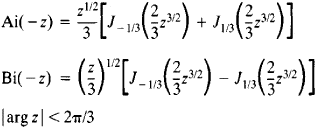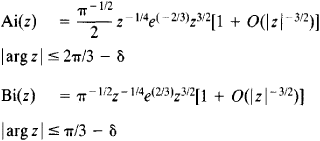Airy Function

(redirected from Airy functions)

Airy function

[¦er·ē ¦fəŋk·shən]
(mathematics)
Either of the solutions of the Airy differential equation.

Airy Function

either of the functions Ai(z) and Bi(z), which are solutions of the second-order differential equation

W″zW = 0

where z is the independent variable.

The Airy functions of the argument (–z) may be expressed in terms of Bessel functions of order v = ±⅓:The asymptotic representations for large |z| areAiry functions play an important role in the theory of asymptotic representations of various special functions; they have diverse applications in mathematical physics—for example, in the theory of the diffraction of radio waves at the earth’s surface. Airy functions were studied by J. R. Airy in 1911.

REFERENCES

Lebedev, N. N. Spetsial’nye funktsii i ikh prilozheniia. 2nd ed. Moscow-Leningrad, 1963.
References in periodicals archive ?
Airy functions, depends on local behavior of the dispersioncurves of individual modes in the vicinity of the corresponding critical points.
The specific form of the wave packet can be finally expressed by using some special functions, slay, in terms of oscillating exponentials, Airy function, Fresnel integral, Pearcey- type integral, etc.
As the 3j, 6j, 9j (angular momenta) and Airy functions chapters were no more dense than the above two, I must have started with the worst
For decades, physicists have known that quantum theory allows for wavelike objects to follow curved trajectories known as Airy functions, after the 19th-century British astronomer George Biddell Airy.
Similar, but thinner shapes, also part of the Airy function, extended parallel to the main parabola, the team reports in the Nov.
Site: Follow: Share:
Open / Close